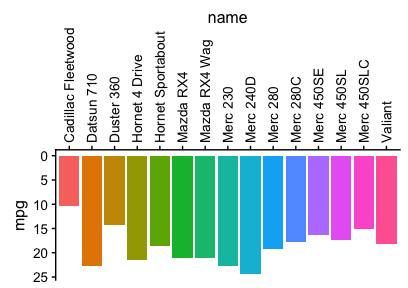# Changing the axis positions

#### 2018-07-15

As of ggplot2 2.2.0, the package now natively supports secondary axes and axes on either side of the plot. Therefore, the cowplot function switch_axis_position() has been deprecated, since it always was an ugly hack and difficult to maintain. Here are some examples of how alternative axes can be created with ggplot2.

require(cowplot)
require(grid) # for unit()
theme_set(theme_cowplot(font_size=12)) # reduce default font size
p1 <- ggplot(mtcars, aes(mpg, disp)) + geom_line(colour = "blue")

# the following code only works for ggplot2 2.2.0 or later
if (packageVersion("ggplot2")>"2.1.0")
p1 + theme_gray() + scale_y_continuous(position = "right")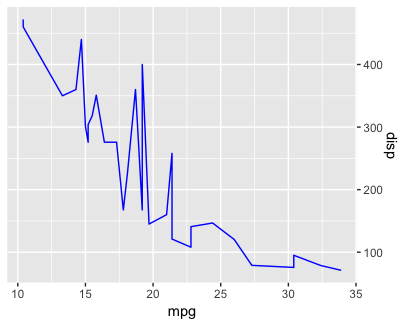if (packageVersion("ggplot2")>"2.1.0")
p1 + theme_bw() + scale_x_continuous(sec.axis = dup_axis())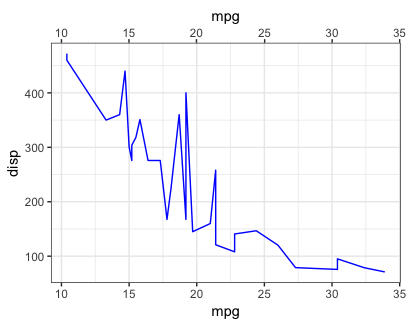if (packageVersion("ggplot2")>"2.1.0")
p1 + scale_x_continuous(sec.axis = dup_axis()) + scale_y_continuous(sec.axis = dup_axis())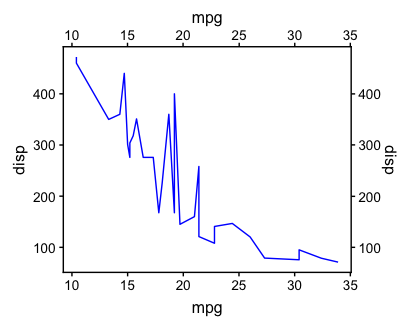if (packageVersion("ggplot2")>"2.1.0")
p1 + theme(axis.ticks.length = unit(0.3, "cm"),
axis.text.x = element_text(margin = margin(0.2, unit = "cm"))) +
scale_x_continuous(sec.axis = dup_axis()) + scale_y_continuous(sec.axis = dup_axis())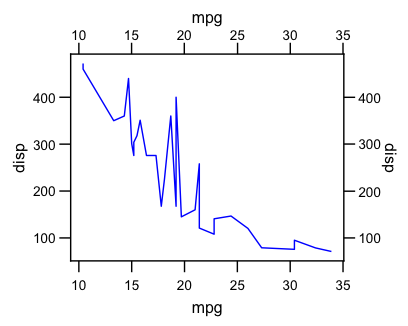It is also possible to use alternate discrete axes:

mtcars2 <- mtcars[1:15, ]
mtcars2\$name <- row.names(mtcars2)

# the following code only works for ggplot2 2.2.0 or later
if (packageVersion("ggplot2")>"2.1.0"){
ggplot(mtcars2, aes(x = name, y = mpg, fill = name)) +
geom_bar(stat = 'identity', position = "identity") +
scale_y_reverse() +
guides(fill = FALSE) +
theme(axis.text.x.top = element_text(angle = 90, vjust=0.5, hjust=0)) +
scale_x_discrete(position = "top")
}